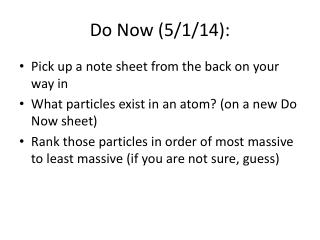# Do Now (5/1/14): - PowerPoint PPT PresentationDownload PresentationDo Now (5/1/14):

Do Now (5/1/14):
Download Presentation## Do Now (5/1/14):

- - - - - - - - - - - - - - - - - - - - - - - - - - - E N D - - - - - - - - - - - - - - - - - - - - - - - - - - -
##### Presentation Transcript

1. Do Now (5/1/14): • Pick up a note sheet from the back on your way in • What particles exist in an atom? (on a new Do Now sheet) • Rank those particles in order of most massive to least massive (if you are not sure, guess)

2. Mass Defect: • The difference between the sum of the mass of the individual nucleon (proton or neutron) and the actual mass.

3. Example: • Find the mass defect of a copper-63 nucleus if the actual mass of a copper-63 nucleus is 62.91367 amu Find the composition of the copper-63 nucleus and determine the combined mass of its components. Copper has 29 protons and copper-63 also has (63 - 29) 34 neutrons. The mass of a proton is 1.00728 amu and a neutron is 1.00867 amu. The combined mass is calculated: 29 protons(1.00728 amu/proton) + 34 neutrons(1.00867 amu/neutron) or 63.50590 amu

4. Example: • Find the mass defect of a copper-63 nucleus if the actual mass of a copper-63 nucleus is 62.91367 amu • Calculate the mass defect. • Dm = 63.50590 amu - 62.91367 amu =  0.59223 amu

5. Binding Energy • The energy equivalent of the mass defect; it is always negative • It is the minimum amount of energy needed to break the nucleus into its component nucleons.

6. Binding Energy of Alpha Particle For the alpha particle Δm= 0.0304 u which gives a binding energy of 28.3 MeV

7. Practice:Work on the Mass Defect worksheet.Finish all mass defect and binding energy problems by the end of class for extra credit!

8. Do Now (5/2/14): • What is the mass defect of an alpha particle if its mass is 4.00153u? • What is the binding energy of an alpha particle?

9. Nuclear Notation

10. Balancing Nuclear Decay Equations

11. Decay Sequence Alpha decay sequence: 235 U 92 4 231 He + Th 2 90 209 Po 84 4 205 He + Pb 2 82

12. Decay Sequence Beta decay sequence: 14 C 6 14 0 N + e 7 -1 228 Ra 88 228 0 Ac + e 89 -1

13. Practice: • Use the rest of class to work on “Mass Defect and Binding Energy” and “Nuclear Reactions.”